# Trigonometry Formulae & Applications (Part 1)Upon request by some students, I’ll discuss a few trigonometry formulae here and also some of their uses in A’levels. I’ve previously discussed the use of factor formulae here under integration.

I’ll start with the R-Formulae. It should require no introduction as it is from secondary Add Math. This formulae is not given in MF26, although students can derive it out using existing formulae in MF26.whereandfor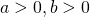and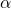is acute.

Here is a quick example,Write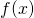as a single trigonometric function exactly.

Here, we observe, we have to use the R-Formulae whereWe have that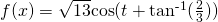.

I’ll end with a question from HCI Midyear 2017 that uses R-Formulae in one part of the question.

A curve D has parametric equations(i) Prove that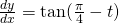.

I’ll discuss about Factor Formulae soon.  And then the difference and application between this two formulae.

Not readable? Change text.